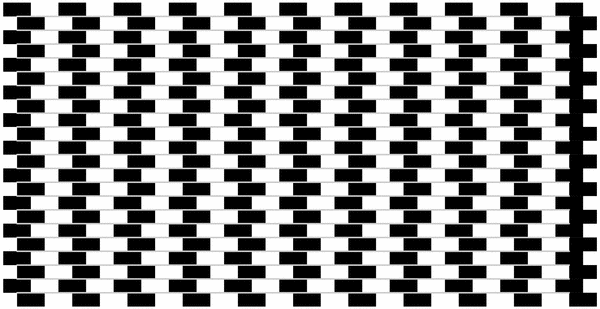Fichier figure001.mp (figure 1) — Modifié le 3 Avril 2008 à 22 h 07Source
``````
input geometriesyr16;

beginfig(1);
path rectangle;
picture rectanglenoir,ligne;
rectangle=(0,0)--u*(1,0)--u*(1,0.5)--u*(0,0.5)--cycle;
rectanglenoir=image(
fill rectangle withcolor noir;
);
for k=0 upto 10:
for j=0 upto 10:
trace rectanglenoir shifted (u*(2*j,k));
endfor;
endfor;
for k=0 upto 10:
for j=0 upto 10:
trace rectanglenoir shifted (u*(2*j-0.5,k+0.5));
endfor;
endfor;
for l=1 upto 21:
trace (0,0.5*u*l)--(20u,0.5*u*l) withpen pencircle scaled 1.5bp withcolor 0.8*white;
endfor;
endfig;

beginfig(2);
trace u*(1,1)--u*(9,1)--u*(9,9)--u*(1,9)--cycle withpen pencircle scaled 2bp;
picture lel,L;
lel=image(
trace
(0,0)--u*(0.5,0)--u*(0.5,0.75)--u*(0.25,0.75)--u*(0.25,0.25)--u*(0,0.25)--cycle
withpen pencircle scaled 1.25bp;
trace u*(0.25,0.25)--u*(0.5,0.25) withpen pencircle scaled 1.25bp;
currentpicture:=currentpicture shifted(u*(2,2));
);
L=image(
for k=-10 upto 10:
for j=-10 upto 15:
trace lel shifted((j*0.5u+k*0.25*u,j*0.25u+k*0.75*u));
endfor;
endfor;
currentpicture:=rotation(currentpicture,iso(u*(2,2),u*(8,8)),-30);